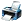Question based on Differential Equation or Application of Derivatives

190 views

If f''(x) = f(x), f(0)=2, f'(0)=3, then find area bounded by the curve f(x) between the axes.

(A)- 3
(B)- 3-5^(0.5)
(C)- 3-0.5xln5
(D)- 2+0.5xln5posted Dec 24, 2017

Simply D^2y-y=0 . Therefore by solving complementary eqn. m^2y-y=0 get the roots m1 and m2 equal to 1 and -1 . The general soln is
Ae^x+Be^-x . Put the values of y=f(0)=2 and f'(0)=0 we get A=5/2 , **strong text
B=-1/2 . Putting y=0 we get x= -0.5ln5 . Taking integration from x=-0.5ln5 to x=0 we get area A=sqrt5-3 . The options given are incorrect .**strong text****answer Mar 24, 2018 by
Hope this will serve your purpose .
Similar Questions